# 自注意力和位置编码2022年10月17日07:34:04

5289字阅读17分37秒

## 自注意力

### 自注意力图解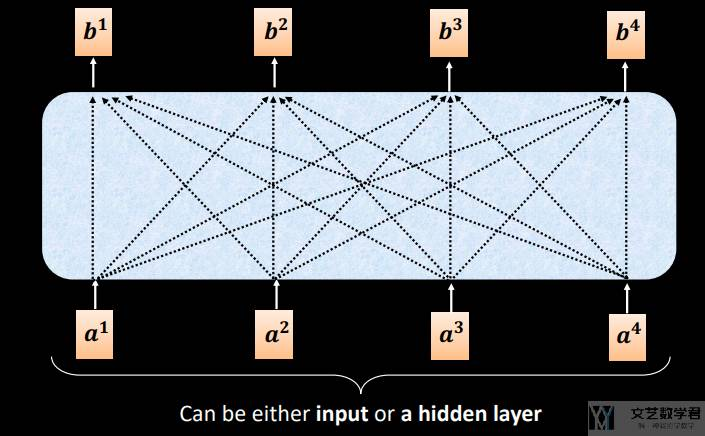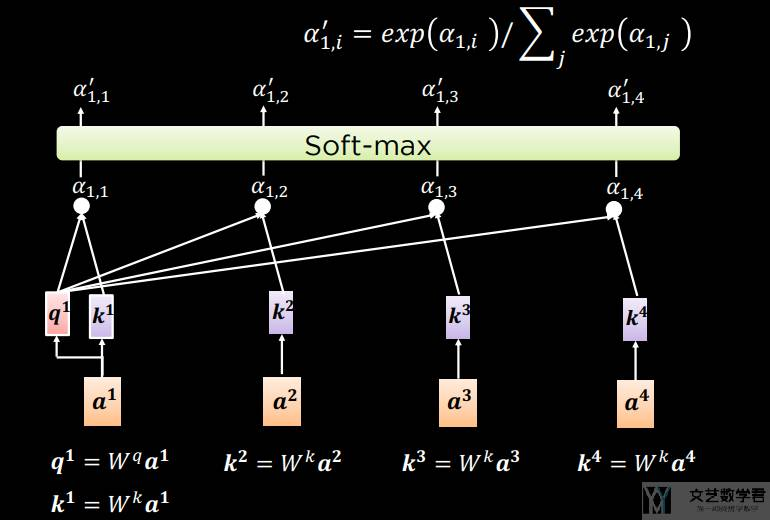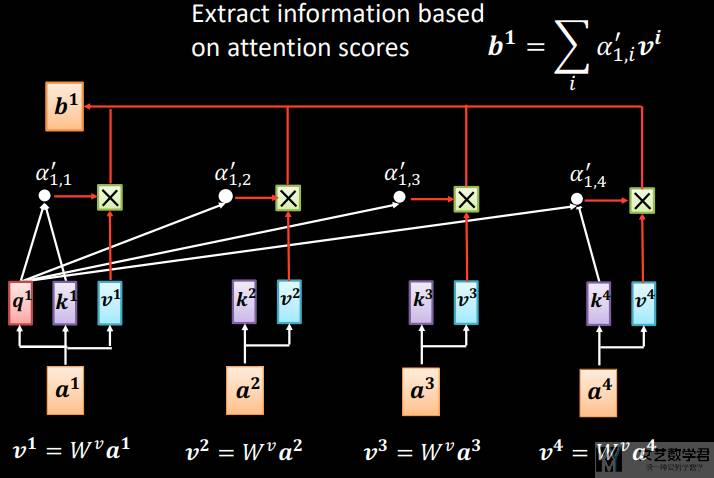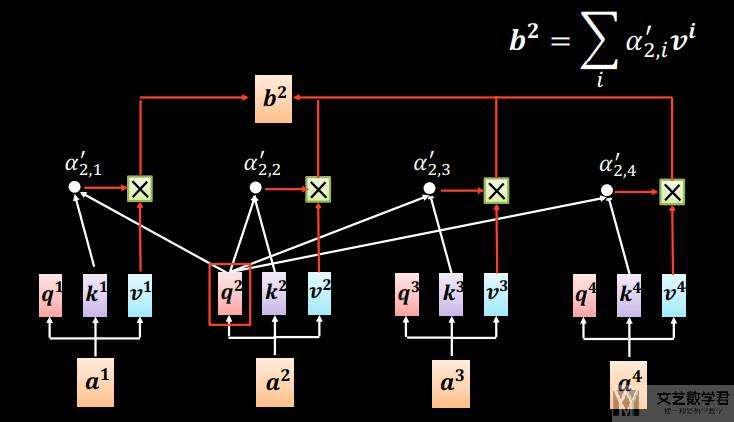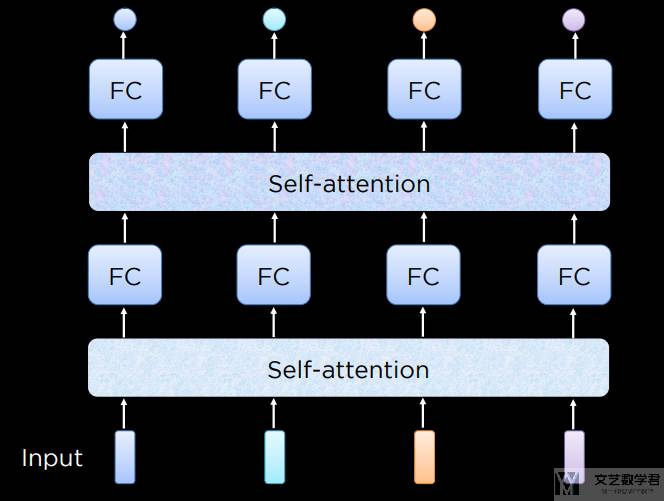### 自注意力并行计算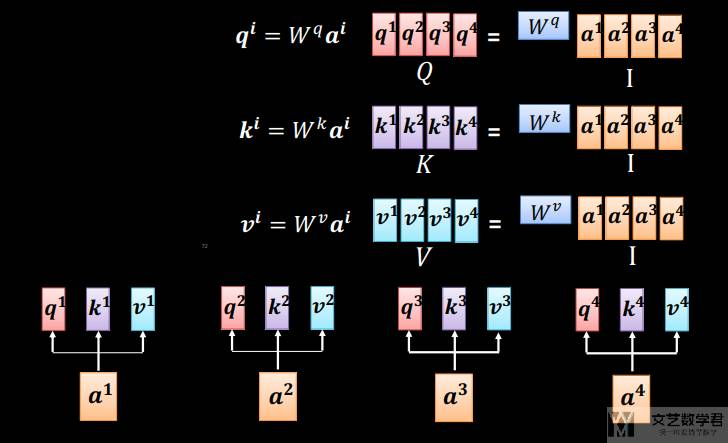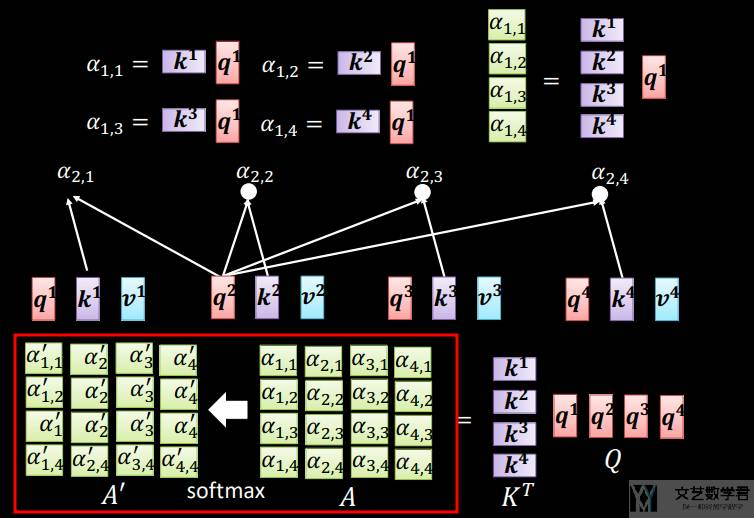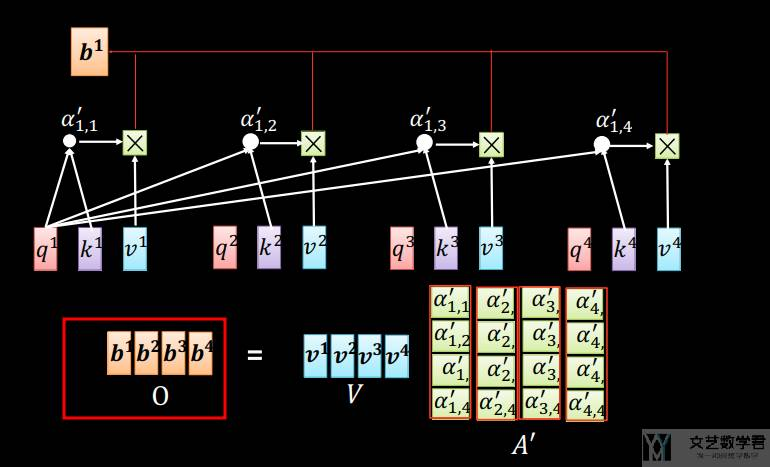### 自注意力数学描述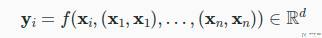### 自注意力代码实现

「自注意力」的代码实现非常简单，就是利用前面的「多头注意力」，唯一不同的就是同样的值，同时作为「查询（`query`）」、「键（`key`）」和「值（`value`）」。

1. # 可以让输入和输出完全一样大小
2. num_hiddens = 100 # `qkv` 转换后的大小维度, 单个的维度为 num_hiddens/num_heads
5. # 构建输入
6. batch_size = 2
7. num_queries = 4
8. valid_lens = torch.tensor([3, 2])
9. X = torch.ones((batch_size, num_queries, num_hiddens))
10. print(X.shape)
11. attention_result = attention(X, X, X, valid_lens) # q, k, v 都是 x
12. # attention 之后的大小 (batch_size, number of queries, num_hiddens)
13. print(attention_result.shape)
14. """
15. torch.Size([2, 4, 100])
16. torch.Size([2, 4, 100])
17. """

1. # 也可以修改输入和输出的大小
2. num_hiddens = 50 # `qkv` 转换后的大小维度, 单个的维度为 num_hiddens/num_heads
5. batch_size = 2
6. num_queries = 4
7. valid_lens = torch.tensor([3, 2])
8. X = torch.ones((batch_size, num_queries, num_hiddens*2))
9. print(X.shape)
10. attention_result = attention(X, X, X, valid_lens) # q, k, v 都是 x
11. print(attention_result.shape) # attention 之后的大小 (batch_size, number of queries, num_hiddens)
12. """
13. X， torch.Size([2, 4, 100])
14. attention_result, torch.Size([2, 4, 50])
15. """

## 位置编码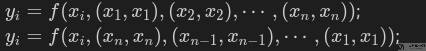1. # 初始化 attention
2. num_hiddens = 10 # `qkv` 转换后的大小维度, 单个的维度为 num_hiddens/num_heads
5. attention.eval()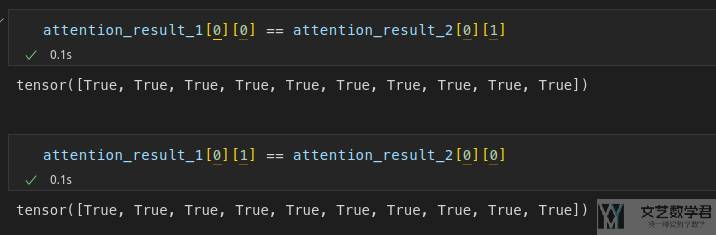### 位置编码想法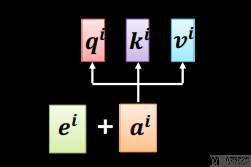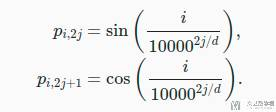• `i` 行，第 `2j` 列，对应第一个式子，也就是 `p_{i, 2j}`
• `i` 行，第 `2j+1` 列，对应第二个式子，也就是 `p_{i, 2j+1}`

### 位置编码实现

1. class PositionalEncoding(nn.Module):
2.     """Positional encoding.
3.     """
4.     def __init__(self, num_hiddens, dropout, max_len=1000):
5.         """初始化位置编码的矩阵
6.         Args:
7.             num_hiddens (int): 每个字转换为多少维度的特征, 相当于有多少列
8.             dropout (float): dropout 的比例, 使得 position encoding 更加鲁棒
9.             max_len (int, optional): 句子的最长的长度, 相当于有多少行. Defaults to 1000.
10.         """
11.         super().__init__()
12.         self.dropout = nn.Dropout(dropout)
13.         # Create a long enough P
14.         self.P = torch.zeros((1, max_len, num_hiddens)) # 初始化一个 max_len*num_hiddens 的矩阵 P
15.         X = torch.arange(max_len, dtype=torch.float32).reshape(-1, 1) # 相当于是 i
16.         Y = torch.pow(10000, torch.arange(0, num_hiddens, 2, dtype=torch.float32) / num_hiddens) # 10000^(2j/d)
17.         X = X/Y # i/(10000^(2j/d))
18.         self.P[:, :, 0::2] = torch.sin(X) # 从 0 开始, 每次间隔 2, 也就是偶数
19.         self.P[:, :, 1::2] = torch.cos(X) # 从 1 开始, 每次间隔 2, 也就是奇数
20.     def forward(self, X):
21.         # 给定 X, 加上 P (位置信息)
22.         X = X + self.P[:, :X.shape, :].to(X.device)
23.         return self.dropout(X)

1. encoding_dim, num_steps = 32, 60
2. pos_encoding = PositionalEncoding(encoding_dim, 0)
3. # 输入大小为 (1, 60, 32), 得到的矩阵大小也是一样
4. X = pos_encoding(torch.zeros((1, num_steps, encoding_dim)))
5. P = pos_encoding.P[:, :X.shape, :] # 只需要与 X 一样的行数
6. plot(
7.     torch.arange(num_steps),
8.     P[0, :, 6:10].T,
9.     xlabel='Row (position)',
10.     figsize=(6, 2.5),
11.     legend=["Col %d" % d for d in torch.arange(6, 10)]
12. )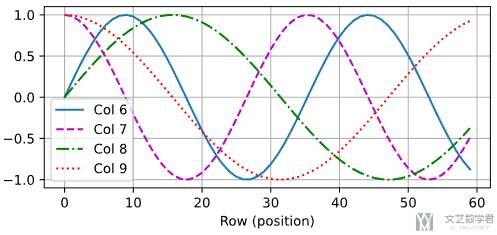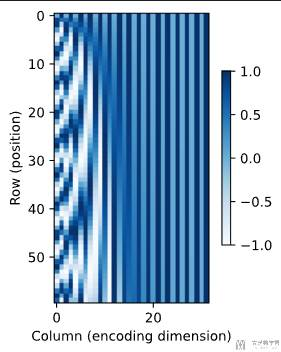### 相对位置信息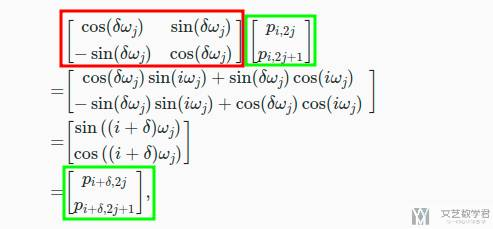• 微信公众号
• 关注微信公众号
•• QQ群
• 我们的QQ群号
•• 本文由 发表于 2022年10月17日07:34:04
• 转载请务必保留本文链接：https://mathpretty.com/15218.html